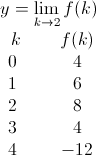# Calculus: Help and Review Final Exam

Free Practice Test Instructions:

Choose your answer to the question and click 'Continue' to see how you did. Then click 'Next Question' to answer the next question. When you have completed the free practice test, click 'View Results' to see your results. Good luck!

#### Question 1 1. What is the derivative of y?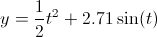#### Question 3 3. Simplify the following expression.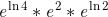#### Question 4 4. If the following graph is an implicit function, then how many real functions would it take to graph it?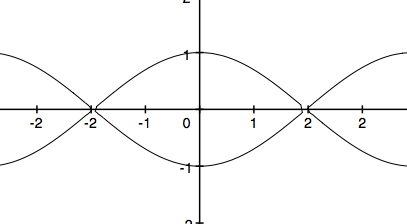#### Question 6 6. Find z, the shaded area in the graph below.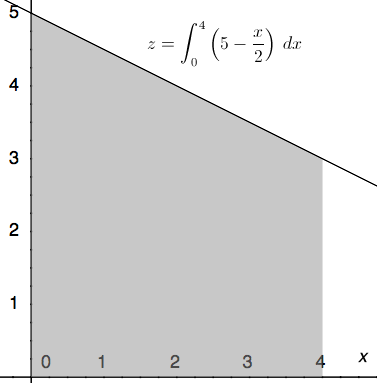#### Question 8 8. What is the volume made by revolving f(x) around the x-axis between x=0 and x=1?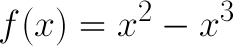#### Question 10 10. Evaluate the integral.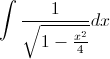#### Question 11 11. What is the derivative of y?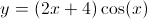#### Question 12 12. Find the derivative of f(x).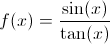#### Question 14 14. What is the highest-order derivative of f(x) that is NOT zero?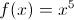#### Question 15 15. Using the given data, solve for the continuous function y.